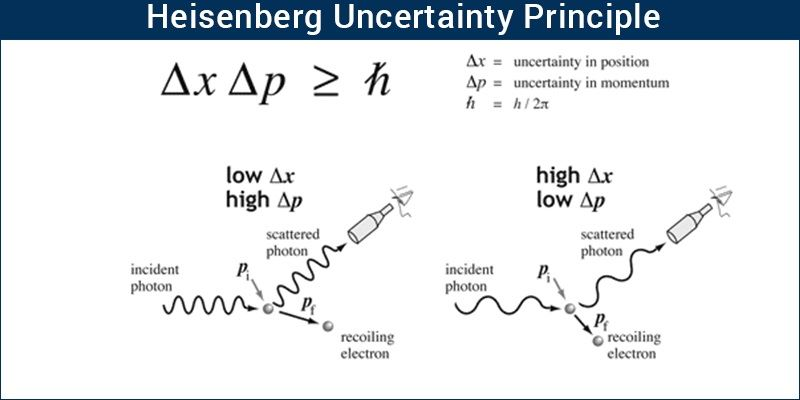# Heisenberg Uncertainty Principle

## What is Heisenberg Uncertainty Principle?

Heisenberg, a German scientist stated the principle of uncertainty which is a consequence of dual nature of matter and waves (De Broglie Relationship). The Heisenberg uncertainty principle states that it is impossible to find the position and momentum (velocity) of an electron at the same moment. Mathematical representation of this is as follows:

△x × △vx ≥ h/4πm

△x is the uncertainty in the position of the electron
△vx is the uncertainty in the momentum (velocity)
h = Planck’s Constant
m = mass

If we know the position of an electron with high accuracy then its velocity will be uncertain. Similarly, if the velocity of an electron is known precisely then its position will be uncertain. The exact picture for both, momentum and position will never be clear. This is the main concept for the principle of uncertainty.Heisenberg uncertainty principle explained

## Importance of Heisenberg Uncertainty Principle

The uncertainty principle rules out the concept of defined paths or trajectories of electrons. The path of an object is determined by its location and velocity at various instants of time. If the exact location of a particle is known along with its velocity at that instant and the forces acting on it then the position of the particle can be determined even after some time. It can be concluded that the position and velocity of a particle and the forces acting on it determine its trajectory.

Since we cannot simultaneously determine the position and velocity of subatomic particles such as electrons, it is impossible to talk about their trajectory. The effect of the Heisenberg uncertainty principle is significant only for the motion of microscopic objects, it is negligible in case of macroscopic objects. For example, we apply the concept of uncertainty to an object of mass 1 milligram.

Know more about Heisenberg uncertainty principle formula.

x×vx = h/4πm

= (6.626×1034JS)/(4×3.1416×106kg)1028m2s1

The value of x×vx is very small and insignificant in this case. Therefore, when we deal with heavier objects the associated uncertainties have no real consequences and can be neglected.

But in the case of microscopic objects like electrons, the value of x×vx is significantly large. For example, the mass of an electron is 9.11 × 10-31 kg and the associated uncertainty is:

x×vx = h/4πm

=(6.626×1034Js)/(4×3.1416×9.11×1031kg)

104m2s1

This means that if we try to find the exact location of an electron, to the uncertainty of 10-8 m, then the uncertainty in velocity will be:

(104m2s1)/(108m) ≈ 104ms1

The uncertainty in velocity is very large and so we cannot claim to find the exact position and velocity of an electron simultaneously. Instead, a probability of momentum and position can be calculated.

We have so far seen the principle of uncertainty and its significance. For any further query kindly install BYJU’S the learning app and have an amazing experience of learning.